# ISEE Middle Level Quantitative : How to multiply fractions

## Example Questions

2 Next →

### Example Question #11 : How To Multiply Fractions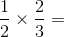Explanation:

When multiplying fractions, simply multiply the numerators by each other and the denominators by each other.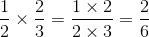Always reduce fractions when possible. In this case, both the numerator and denominator are divisible by 2.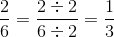### Example Question #12 : How To Multiply Fractions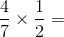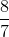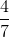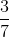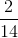Explanation:

When multiplying fractions, multiply the numerators and denominators.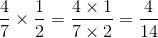When possible, always reduce your fraction. In this case, both 4 and 14 are divisible by 2.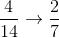### Example Question #171 : Fractions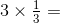Explanation:

When dealing with fractions and whole numbers, first convert the whole number into a fraction. This is easily done by putting the whole number over 1.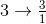When multiplying fractions, multiply both the numerators and denominators.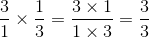When possible, always reduce your fraction. In this case, since the numerator and denominator is the same, the result is 1.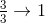### Example Question #14 : How To Multiply Fractions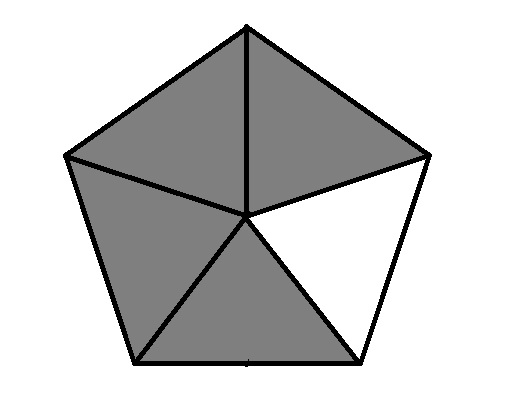The above pentagon has area 100. Which is the greater quantity?

(a) The area of the white region

(b) 25

It is impossible to determine which is greater from the information given

(a) is the greater quantity

(a) and (b) are equal

(b) is the greater quantity

(b) is the greater quantity

Explanation:

Of the five triangles of equal area, one of them is white - this isof the pentagon. The pentagon has area 100, so the area of the white triangle is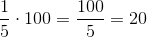,

which is less than 25.

### Example Question #15 : How To Multiply Fractions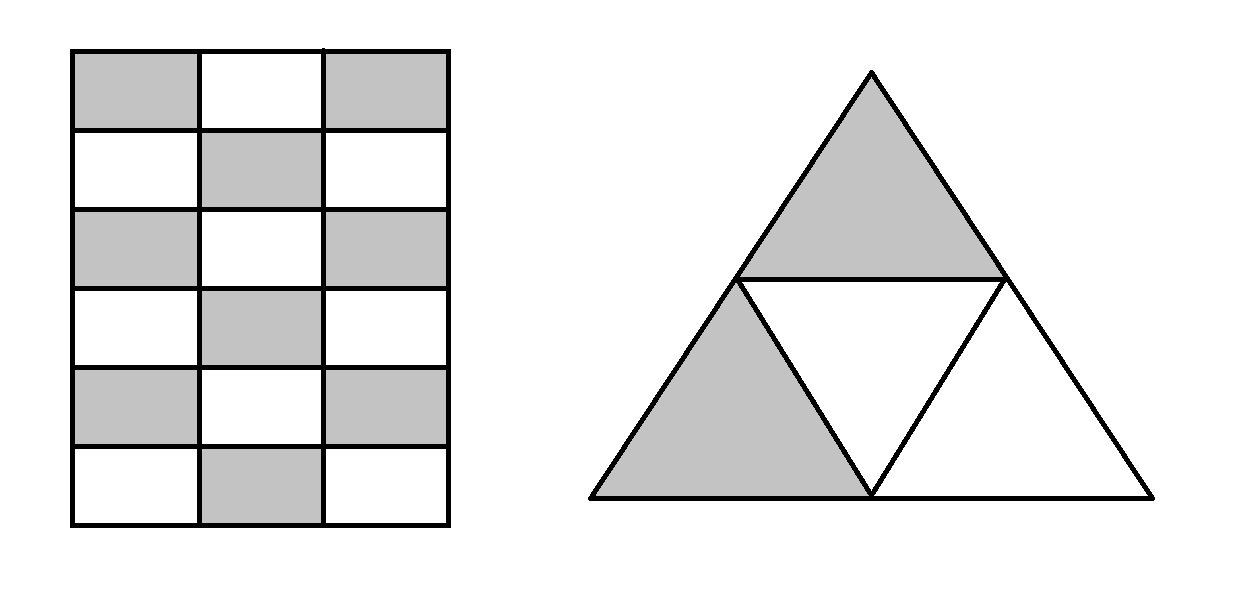Note: figure NOT drawn to scale

Above are a rectangle and a triangle, each of which is divided equally into smaller shapes. Which is the greater quantity?

(a) The area of the shaded portion of the rectangle

(b) The area of the shaded portion of the triangle

(a) is the greater quantity

(b) is the greater quantity

It is impossible to determine which is greater from the information given

(a) and (b) are equal